### LEXICO Statement

(Experimental)

LEXICO lexicographic_ordering_constraint-1 <…lexicographic_ordering_constraint-n> ;

LEXORDER lexicographic_ordering_constraint-1 <…lexicographic_ordering_constraint-n> ;

where lexicographic_ordering_constraint is specified in the following form:

((variable-list-1) order_type (variable-list-2))

where variable-list-1 and variable-list-2 are variable lists of equal length. The keyword order_type signifies the type of ordering and can be one of two values: LEX_LE, which indicates lexicographically less than or equal to (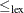), or LEX_LT, which indicates lexicographically less than (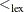).

The LEXICO statement specifies one or more lexicographic constraints. The lexicographic constraintand the strict lexicographic constraintare defined as follows. Given two-tuples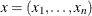and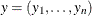, the-tupleis lexicographically less than or equal to
(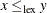) if and only if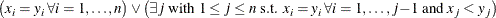The-tupleis lexicographically less than(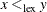) if and only ifand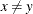. Equivalently,if and only if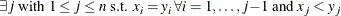Informally you can think of the lexicographic constraintas sorting the-tuples in alphabetical order. Mathematically,is a partial order on a given subset of-tuples, andis a strict partial order on a given subset of-tuples (Brualdi, 2010).

For example, you can express the lexicographic constraint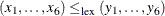by using a LEXICO statement as follows:

lexico( (x1-x6) lex_le (y1-y6) );


The assignment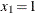,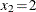,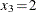,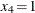,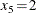,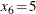,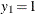,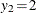,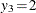,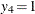,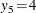, and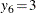satisfies this constraint because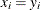for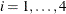and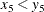. The fact that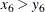is irrelevant in this ordering.

Lexicographic ordering constraints can be useful for breaking a certain kind of symmetry that arises in CSPs with matrices of decision variables. Frisch et al. (2002) introduce an optimal algorithm to establish GAC (generalized arc consistency) for theconstraint between two vectors of variables.